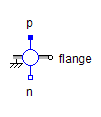Single Phase 2 Level - MapleSim Help

Single Phase 2 Level

Single phase DC to AC converterDescription The Single Phase 2 Level component models the output driver of a single-phase inverter. It consists of two transistors in series, each with a parallel free-wheeling diode. The transistors are enabled by the boolean inputs ${\mathrm{fire}}_{p}$ and ${\mathrm{fire}}_{n}$. To see an example model that uses this component, search for SinglePhase2Level in the MapleSim search bar.Connections

 Name Description Modelica ID ${\mathrm{dc}}_{p}$ Positive DC input dc_p ${\mathrm{dc}}_{n}$ Negative DC input dc_n $\mathrm{ac}$ AC output ac $\mathrm{heatPort}$ Conditional heat port heatPort $\mathrm{enable}$ Boolean input; enables fire ${\mathrm{fire}}_{p}$ and ${\mathrm{fire}}_{n}$ enable ${\mathrm{fire}}_{p}$ Boolean input; firing signal of positive potential transistor fire_p ${\mathrm{fire}}_{n}$ Boolean input; firing signal of negative potential transistor fire_nParametersGeneral Parameters

 Name Default Units Description Modelica ID ${R}_{\mathrm{on}\left(\mathrm{thyristor}\right)}$ $1·{10}^{-5}$ $\mathrm{\Omega }$ Transistor closed resistance RonTransistor ${G}_{\mathrm{off}\left(\mathrm{thyristor}\right)}$ $1·{10}^{-5}$ $S$ Transistor opened conductance GoffTransistor ${V}_{\mathrm{knee}\left(\mathrm{thyristor}\right)}$ $0$ $V$ Transistor threshold voltage VkneeTransistor ${R}_{\mathrm{on}\left(\mathrm{diode}\right)}$ $1·{10}^{-5}$ $\mathrm{\Omega }$ Diode closed resistance RonDiode ${G}_{\mathrm{off}\left(\mathrm{diode}\right)}$ $1·{10}^{-5}$ $S$ Diode opened conductance GoffDiode ${V}_{\mathrm{knee}\left(\mathrm{diode}\right)}$ $0$ $V$ Diode threshold voltage VkneeDiode Use Heat Port $\mathrm{false}$ True (checked) means heat port is enabled useHeatPortEnable Parameters

 Name Default Units Description Modelica ID Use Constant Enable $\mathrm{true}$ True (checked) means enable the Constant Enable parameter; false means enable a boolean input. useConstantEnable Constant Enable $\mathrm{true}$ True (checked) means enable the firing signal; false means disable the firing signal constantEnableModelica Standard Library The component described in this topic is from the Modelica Standard Library. To view the original documentation, which includes author and copyright information, click here.